# Plotting¶

This chapter presents ways to create figures and graphs, more generally called data visualizations. As examples, we’ll generate three figures:

• We’ll replicate a figure from the Pew Research Center that shows changes in religious affiliation in the U.S. over time.

• We’ll replicate the figure from The Economist that shows the prices of sandwiches in Boston and London (we saw this data back in Chapter 3).

• We’ll make a plot to test Zipf’s law, which describes the relationship between word frequencies and their ranks.

With the tools in this chapter, you can generate a variety of simple graphs. We will see more visualization tools in later chapters.

But before we get started with plotting, we need a new language feature: keyword arguments.

## Keyword arguments¶

When you call most functions, you have to provide values. For example, when you call np.exp, the value you provide is a number:

import numpy as np

np.exp(1)

2.718281828459045


When you call np.power, you have to provide two numbers:

np.power(10, 6)

1000000


The values you provide are called arguments. Specifically, the values in these examples are positional arguments because their position determines how they are used. In the second example, power computes 10 to the sixth power, not 6 to the 10th power because of the order of the arguments.

Many functions also take keyword arguments, which are optional. For example, we have previously used int to convert a string to an integer.

int('21')

21


By default, int assumes that the number is in base 10. But you can provide a keyword argument that specifies a different base. For example, the string '21', interpreted in base 8, represents the number 2 * 8 + 1 = 17. Here’s how we do this conversion using int.

int('21', base=8)

17


The string '21' is a positional argument. The integer value 8 is a keyword argument, with the keyword base.

Specifying a keyword argument looks like an assignment statement, but it does not create a new variable. And when you specify a keyword argument, you don’t choose the variable name. In this example, the keyword name, base, is part of the definition of int. If you specify another keyword name, you get an error.

Exercise: The print function takes a keyword argument called end that specifies the character it prints at the end of the line. By default, end is the newline character, \n. So if you call print more than once, the results normally appear on separate lines, like this:

for x in [1, 2, 3]:
print(x)

1
2
3


Modify the previous example so it prints the elements of the list, all on one line, with spaces between them. Then modify it to print an open bracket at the beginning and a close bracket and newline at the end.

## Religious affiliation¶

Now we’re ready to make some graphs.

In October 2019 the Pew Research Center published “In U.S., Decline of Christianity Continues at Rapid Pace” at https://www.pewforum.org/2019/10/17/in-u-s-decline-of-christianity-continues-at-rapid-pace. It includes this figure, which shows changes in religious affiliation among adults in the U.S. over the previous 10 years.As an exercise, we’ll replicate this figure. It shows results from two sources, Religious Landscape Studies and Pew Research Political Surveys. The political surveys provide data from more years, so we’ll focus on that.

The data from the figure are available from Pew Research at https://www.pewforum.org/wp-content/uploads/sites/7/2019/10/Detailed-Tables-v1-FOR-WEB.pdf, but they are in a PDF document. It is sometimes possible to extract data from PDF documents, but for now we’ll enter the data by hand.

year = [2009, 2010, 2011, 2012, 2013, 2014, 2015, 2016, 2017, 2018]

christian = [77, 76, 75, 73, 73, 71, 69, 68, 67, 65]

unaffiliated = [17, 17, 19, 19, 20, 21, 24, 23, 25, 26]


The library we’ll use for plotting is Matplotlib; more specifically, we’ll use a part of it called Pyplot, which we’ll import with the nickname plt.

import matplotlib.pyplot as plt


Pyplot provides a function called plot that makes a line plot. It takes two sequences as arguments, the x values and the y values. The sequences can be tuples, lists, or arrays.

plt.plot(year, christian);

[<matplotlib.lines.Line2D at 0x7fe71a051e50>]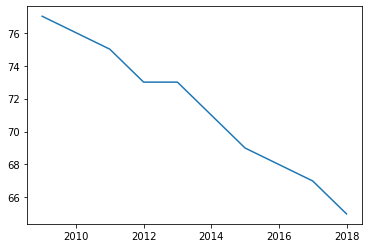The semi-colon at the end of the line cleans up the output; without it, the return value from plot would be displayed above the figure.

If you plot multiple lines in a single cell, they appear on the same axes.

plt.plot(year, christian)
plt.plot(year, unaffiliated);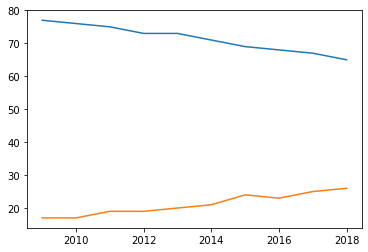Plotting them on the same axes makes it possible to compare them directly. However, notice that Pyplot chooses the range for the axes automatically; in this example the y axis starts around 15, not zero.

As a result, it provides a misleading picture, making the ratio of the two lines look bigger than it really is. We can set the limits of the y axis using the function plt.ylim. The argument is a list with two values, the lower bound and the upper bound.

plt.plot(year, christian)
plt.plot(year, unaffiliated)

plt.ylim([0, 80]);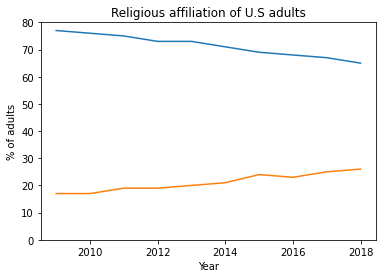That’s better, but this graph is missing some of the most important elements: labels for the axes and a title.

## Decorating the axes¶

To label the axes and add a title, we’ll use Pyplot functions xlabel, ylabel, and title. All of them take strings as arguments.

plt.plot(year, christian)
plt.plot(year, unaffiliated)

plt.ylim([0, 80])
plt.xlabel('Year')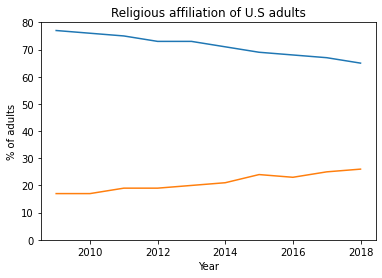Now let’s add another important element, a legend that indicates which line is which. To do that, we add a label to each line, using the keyword argument label. Then we call plt.legend to create the legend.

plt.plot(year, christian, label='Christian')
plt.plot(year, unaffiliated, label='Unaffiliated')

plt.ylim([0, 80])
plt.xlabel('Year')
plt.legend();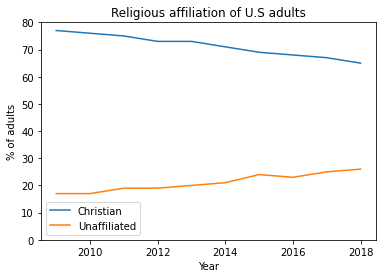Exercise: The orginal figure plots lines between the data points, but it also plots “markers” showing the location of each data point. It is generally good practice to include markers, especially if data are not available for every year.

Modify the previous example to include a keyword argument marker with the string value 'o', which indicates that you want to plot circles as markers.

Exercise: In the original figure, the line labelled 'Christian' is red and the line labeled 'Unaffiliated' is grey.

Find the online documentation of plt.plot and figure out how to use keyword arguments to specify colors. Choose colors to (roughly) match the original figure.

The legend function takes a keyword argument that speficies the location of the legend. Read the documentation of this function and move the legend to the center left of the figure.

## Sandwiches¶

In a previous chapter we used data from an article in The Economist comparing sandwich prices in Boston and London: “Why Americans pay more for lunch than Britons do” at https://www.economist.com/finance-and-economics/2019/09/07/why-americans-pay-more-for-lunch-than-britons-do.

The article includes this graph showing prices of several sandwiches in the two cities:As an exercise, let’s see if we can replicate this figure. Here’s the data from the article again: the names of the sandwiches and the price list for each city.

name_list = [
'Lobster roll',
'Chicken caesar',
'Bang bang chicken',
'Ham and cheese',
'Tuna and cucumber',
'Egg'
]

boston_price_list = [9.99, 7.99, 7.49, 7, 6.29, 4.99]
london_price_list = [7.5, 5, 4.4, 5, 3.75, 2.25]


In the previous section we plotted percentages on the y axis versus time on the x axis. Now we want to plot the sandwich names on the y axis and the prices on the x axis. Here’s how:

plt.plot(boston_price_list, name_list)
plt.xlabel('Price in USD');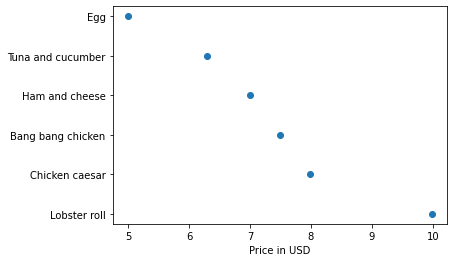name_list is a list of strings; Pyplot orders them from top to bottom, equally spaced.

By default Pyplot connects the points with lines, but in this example the lines don’t make sense because the sandwich names are categorical, not numerical. You can’t interpolate between an egg sandwich and a tuna sandwich.

We can turn on markers and turn off lines with keyword arguments.

plt.plot(boston_price_list, name_list,
marker='o', linestyle='')
plt.xlabel('Price in USD');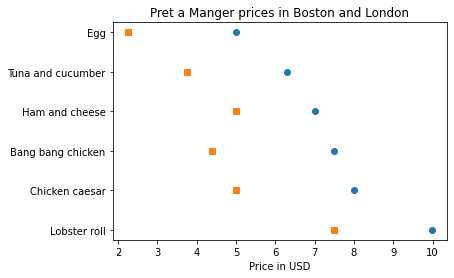Or we can do the same thing more concisely by providing a format string as a positional argument. You can read the documentation of plt.plot to learn more about format strings.

plt.plot(boston_price_list, name_list, 'o')
plt.plot(london_price_list, name_list, 's')

plt.xlabel('Price in USD')
plt.title('Pret a Manger prices in Boston and London');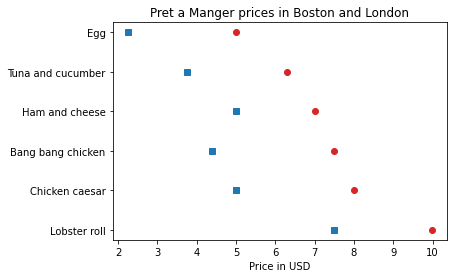I added a title at the same time.

Now, to approximate the colors in the original figure, we can use the strings 'C3' and 'C0', which specify colors from the default color sequence. You can read the documentation to learn more about specifying colors in Pyplot: https://matplotlib.org/3.1.1/tutorials/colors/colors.html.

plt.plot(boston_price_list, name_list, 'o', color='C3')
plt.plot(london_price_list, name_list, 's', color='C0')

plt.xlabel('Price in USD')
plt.title('Pret a Manger prices in Boston and London');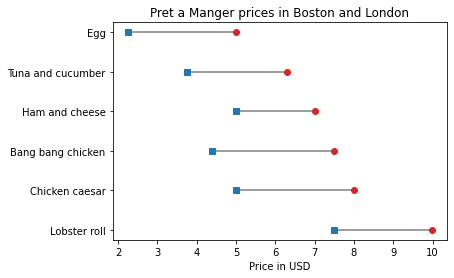To connect the dots with lines, we’ll use plt.hlines, which draws horizontal lines. It takes three arguments: a sequence of values on the y axis, which are the sandwich names in this example, and two sequences of values on the x axis, which are the London prices and Boston prices.

plt.plot(boston_price_list, name_list, 'o', color='C3')
plt.plot(london_price_list, name_list, 's', color='C0')

plt.hlines(name_list, london_price_list, boston_price_list)

plt.xlabel('Price in USD')
plt.title('Pret a Manger prices in Boston and London');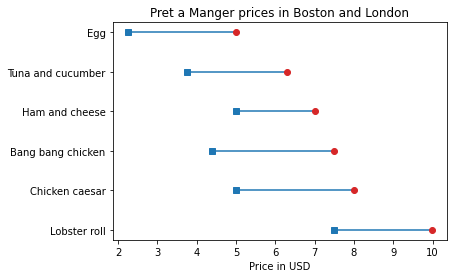Exercise: To finish off this example, add a legend that identifies the London and Boston prices. Remember that you have to add a label keyword each time you call plt.plot, and then call plt.legend.

Notice that the sandwiches in our figure are in the opposite order of the sandwiches in the original figure. There is a Pyplot function that inverts the y axis; see if you can find it and use it to reverse the order of the sandwich list.

## Zipf’s law¶

In the previous chapter we downloaded War and Peace from Project Gutenberg and counted the number of lines and words. Then we used a dictionary to count the number of unique words and the number of times each one appears. Now we’ll use those results to generate a “Zipf plot”, which shows the frequency of the words on the y axis, ordered from the most common word to the least.

In the previous chapter, we looped through the book and made a string that contains all punctuation characters. Here are the results, which we will need again.

all_punctuation = ',.-:[#]*/“’—‘!?”;()%@'


And here’s a solution to one of the previous exercises. It loops through the book and makes a dictionary that maps from each word to the number of times it appears.

first_line = "CHAPTER I\n"
last_line = ("End of the Project Gutenberg EBook of " +
"War and Peace, by Leo Tolstoy\n")

fp = open('2600-0.txt')
for line in fp:
if line == first_line:
break

unique_words = {}
for line in fp:
if line == last_line:
break

for word in line.split():
word = word.lower()
word = word.strip(all_punctuation)
if word in unique_words:
unique_words[word] += 1
else:
unique_words[word] = 1


## Frequencies and ranks¶

In this section we’ll test Zipf’s law, which states that

given some corpus of natural language utterances, the frequency of any word is inversely proportional to its rank in the frequency table. Thus the most frequent word will occur approximately twice as often as the second most frequent word, three times as often as the third most frequent word, etc.

See https://en.wikipedia.org/wiki/Zipfs_law. To see if this law holds for the words in War and Peace, we’ll make a plot that shows:

• The frequency of each word on the y axis, and

• The rank of each word on the x axis, where the rank of the most frequent word is 1, the rank of the second most common word is 2, etc.

In unique_words, the keys are words and the values are their frequencies. We can use the values function to get the values from the dictionary. The result has the type dict_values:

freqs = unique_words.values()
type(freqs)

dict_values


Before we plot them, we have to sort them, but the sort function doesn’t work with dict_values.

We can use list to make a list of frequencies:

freqs = list(unique_words.values())
type(freqs)

list


And now we can use sort. By default it sorts in ascending order, but we can pass a keyword argument to reverse the order.

freqs.sort(reverse=True)


Now, for the ranks, we need a sequence that counts from 1 to n, where n is the number of elements in freqs. We can use the range function, which returns a value with type range.

As a small example, here’s the range from 1 to 5.

range(1, 5)

range(1, 5)


However, there’s a catch. If we use the range to make a list, we see that “the range from 1 to 5” includes 1, but it doesn’t include 5.

list(range(1, 5))

[1, 2, 3, 4]


That might seem strange, but it is often more convenient to use range when it is defined this way, rather than what might seem like the more natural way (see https://www.cs.utexas.edu/users/EWD/transcriptions/EWD08xx/EWD831.html). Anyway, we can get what we want by increasing the second argument by one:

list(range(1, 6))

[1, 2, 3, 4, 5]


So, finally, we can make a range that represents the ranks from 1 to n:

n = len(freqs)
ranks = range(1, n+1)
ranks

range(1, 20479)


And now we can plot the frequencies versus the ranks:

plt.plot(ranks, freqs)

plt.xlabel('Rank')
plt.ylabel('Frequency')
plt.title("War and Peace and Zipf's law");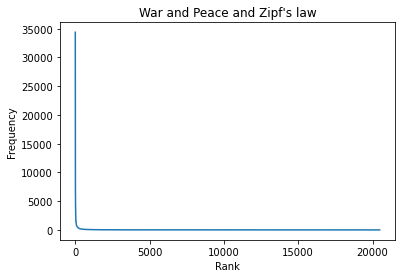## Logarithmic scales¶

The few most common words are very common, but the great majority of words are rare. So that’s consistent with Zipf’s law, but Zipf’s law is more specific. It claims that the frequencies should be inversely proportional to the ranks. If that’s true, we can write:

$$f = k / r$$

where $$r$$ is the rank of a word, $$f$$ is its frequency, and $$k$$ is an unknown constant of proportionality. If we take the log of both sides, we get this:

$$\log f = \log k - \log r$$

This equation implies that if we plot $$f$$ versus $$r$$ on a log-log scale, we expect to see a straight line with intercept at $$\log k$$ and slope -1.

We can use plt.xscale to plot the x axis on a log scale.

plt.plot(ranks, freqs)

plt.xlabel('Rank')
plt.ylabel('Frequency')
plt.title("War and Peace and Zipf's law")
plt.xscale('log')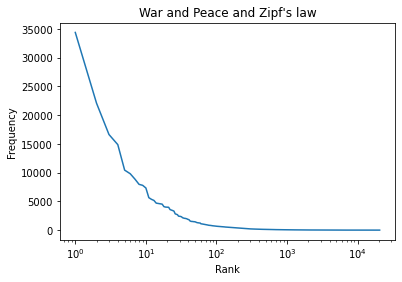And plt.yscale to plot the y axis on a log scale.

plt.plot(ranks, freqs)

plt.xlabel('Rank')
plt.ylabel('Frequency')
plt.title("War and Peace and Zipf's law")
plt.xscale('log')
plt.yscale('log')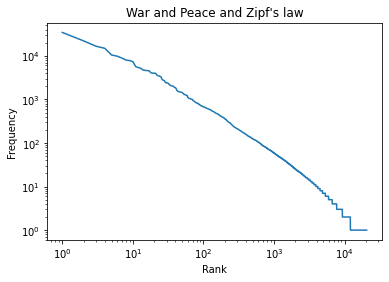The result is not quite a straight line, but it is close. We can get a sense of the slope by connecting the end points with a line. I’ll select the first and last elements from xs.

xs = ranks, ranks[-1]
xs

(1, 20478)


And the first and last elements from ys.

ys = freqs, freqs[-1]
ys

(34388, 1)


And plot a line between them.

plt.plot(xs, ys, color='gray')
plt.plot(ranks, freqs)

plt.xlabel('Rank')
plt.ylabel('Frequency')
plt.title("War and Peace and Zipf's law")
plt.xscale('log')
plt.yscale('log')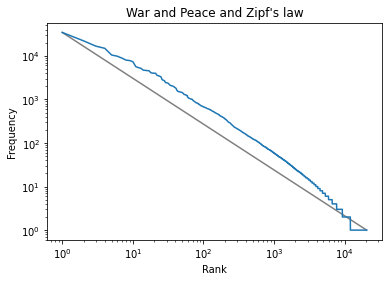The slope of this line is the “rise over run”, that is, the difference on the y axis divided by the difference on the x axis.

We can compute the rise using np.log10 to compute the log base 10 of the first and last values:

np.log10(ys)

array([4.53640692, 0.        ])


Then we can use np.diff to compute the difference between the elements:

rise = np.diff(np.log10(ys))
rise

array([-4.53640692])


Exercise: Use log10 and diff to compute the run, that is, the difference on the x axis. Then divide the rise by the run to get the slope of the grey line. Is it close to -1, as Zipf’s law predicts?